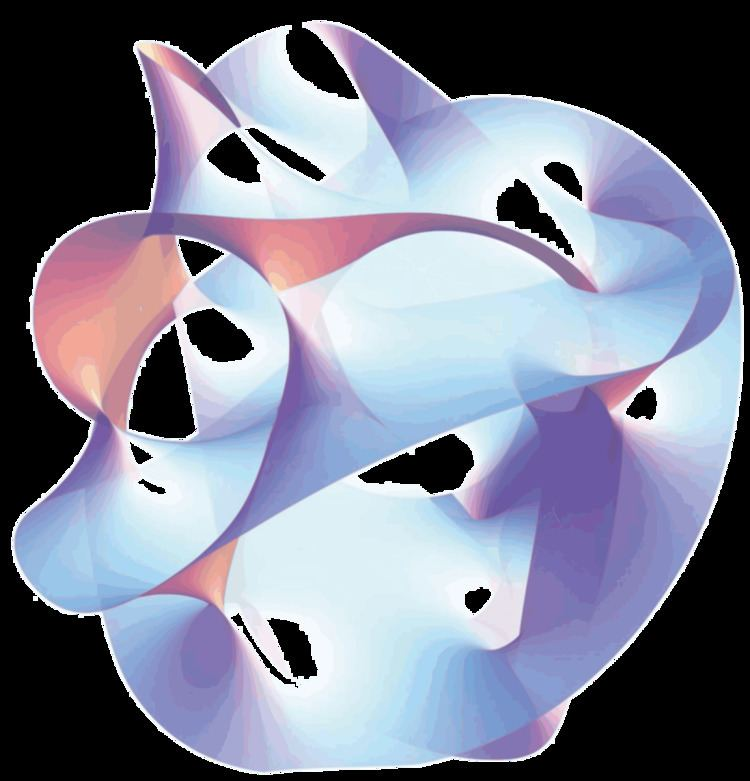# Matrix theory (physics)

Updated on
Covid-19In theoretical physics, the BFSS matrix model or matrix theory is a quantum mechanical model proposed by Tom Banks, Willy Fischler, Stephen Shenker, and Leonard Susskind in 1997.

## Overview

This theory describes the behavior of a set of nine large matrices. In their original paper, these authors showed, among other things, that the low energy limit of this matrix model is described by eleven-dimensional supergravity. These calculations led them to propose that the BFSS matrix model is exactly equivalent to M-theory. The BFSS matrix model can therefore be used as a prototype for a correct formulation of M-theory and a tool for investigating the properties of M-theory in a relatively simple setting. The BFSS matrix model is also considered the worldvolume theory of a large number of D0-branes in type IIA string theory.

## Noncommutative geometry

In geometry, it is often useful to introduce coordinates. For example, in order to study the geometry of the Euclidean plane, one defines the coordinates x and y as the distances between any point in the plane and a pair of axes. In ordinary geometry, the coordinates of a point are numbers, so they can be multiplied, and the product of two coordinates does not depend on the order of multiplication. That is, xy = yx. This property of multiplication is known as the commutative law, and this relationship between geometry and the commutative algebra of coordinates is the starting point for much of modern geometry.

Noncommutative geometry is a branch of mathematics that attempts to generalize this situation. Rather than working with ordinary numbers, one considers some similar objects, such as matrices, whose multiplication does not satisfy the commutative law (that is, objects for which xy is not necessarily equal to yx). One imagines that these noncommuting objects are coordinates on some more general notion of "space" and proves theorems about these generalized spaces by exploiting the analogy with ordinary geometry.

In a paper from 1998, Alain Connes, Michael R. Douglas, and Albert Schwarz showed that some aspects of matrix models and M-theory are described by a noncommutative quantum field theory, a special kind of physical theory in which the coordinates on spacetime do not satisfy the commutativity property. This established a link between matrix models and M-theory on the one hand, and noncommutative geometry on the other hand. It quickly led to the discovery of other important links between noncommutative geometry and various physical theories.

## Related models

Another notable matrix model capturing aspects of Type IIB string theory, the IKKT matrix model, was constructed in 1996–97 by N. Ishibashi, H. Kawai, Y. Kitazawa, A. Tsuchiya.

## References

Similar Topics
Twilight Online
Josep Ferrer Bujons
Dani Ponce
Topics